# Important Questions Class 10 Maths Chapter 11 - Constructions

Important questions for Class 10 Maths Chapter 11 Constructions are provided here based on the new pattern of CBSE. Students preparing for the Class 10 board exams 2022-2023 can practice these questions of Construction For Class 10 to score full marks for the questions from this chapter. These important questions will be helpful for the students to answer the questions on this chapter in the class 10 Maths paper.

The chapter Constructions deals with the construction of line segments, construction of similar triangles with the given scale factor and the construction of tangents to the circle. Students can also refer to these important questions as a part of their board exam preparation. NCERT Solutions are also available at BYJU’S to help the students in scoring full marks.

Students can also get access to Class 10 Maths Chapter 11 Constructions MCQs here.

## Important Questions & Answers For Class 10 Maths Chapter 11 Constructions

Students can have a look at the CBSE Class 10 Maths Important Questions Chapter 11 on Construction and their answers below. For more practice, we have also provided a few more questions at the end of this page.

Q.1: Draw a line segment of length 7 cm. Find a point P on it which divides it in the ratio 3:5.
Solution:
Steps of construction:
Step 1: Draw a line segment, AB = 7 cm.
Step 2: Draw a ray, AX, making an acute angle downward with AB.
Step 3: Mark the points A1, A2, A3 … A8 on AX.
Step 4: Mark the points such that AA1 = A1A2 = A2A3 = ….., A7A8.
Step 5: Join BA8.
Step 6: Draw a line parallel to BA8 through the point A3, to meet AB on P.
Hence AP: PB = 3: 5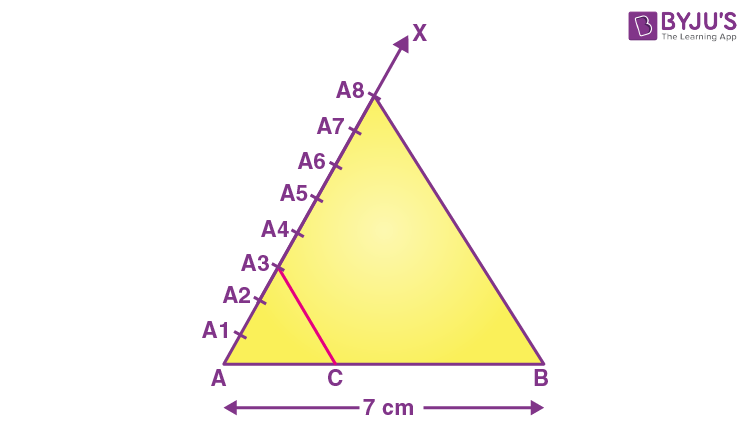Q.2: Construct a triangle with sides 5 cm, 6 cm and 7 cm and then another triangle whose sides are 7/5 of the corresponding sides of the first triangle.
Solution:

Steps of Construction:

Step 1: Draw a line segment AB =5 cm.

Step 2: Take A and B as centre, and draw the arcs of radius 6 cm and 7 cm respectively.

Step 3: These arcs will intersect each other at point C, and therefore ΔABC is the required triangle with the length of sides as 5 cm, 6 cm, and 7 cm respectively.

Step 4: Draw a ray AX which makes an acute angle with the line segment AB on the opposite side of vertex C.

Step 5: Locate the 7 points such as A1, A2, A3, A4, A5, A6, A7 (as 7 is greater between 5and 7), on line AX such that it becomes AA1 = A1A2 = A2A3 = A3A4 = A4A5 = A5A6 = A6A7.

Step 6: Join the points BA5 and draw a line from A7 to BA5 which is parallel to the line BA5 where it intersects the extended line segment AB at point B’.

Step 7: Now, draw a line from B’ the extended line segment AC at C’ which is parallel to the line BC and it intersects to make a triangle.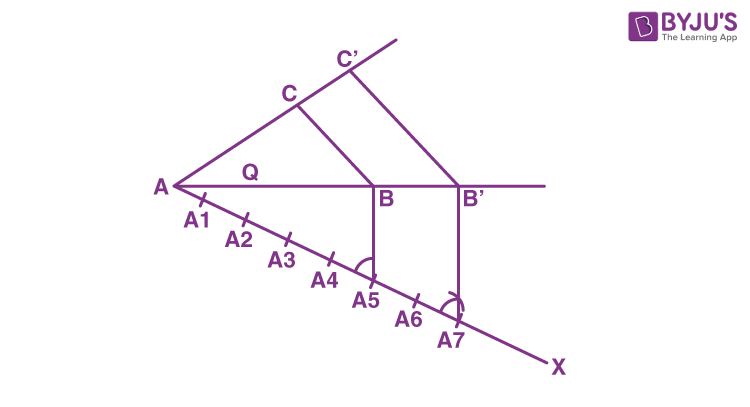Therefore, ΔAB’C’ is the required triangle.

Q.3: Draw a circle of radius 3 cm. Take two points P and Q on one of its extended diameters, each at a distance of 7 cm from its centre. Draw tangents to the circle from these two points P and Q.

Solution:

Steps of construction:

Step 1: Draw a circle with a radius of 3 cm with centre “O”.

Step 2: Draw a diameter of a circle with endpoints P and Q, and it extends 7 cm from the centre.

Step 3: Draw the perpendicular bisector of the line PO and mark the midpoint as M.

Step 4: Draw a circle with M as centre and MO as the radius

Step 5: Now join the points PA and PB in which the circle with radius MO intersects the circle at points A and B.

Step 6: Now PA and PB are the required tangents.

Step 7: Similarly, from point Q, we can draw the tangents.

Step 8: From that, QC and QD are the required tangents.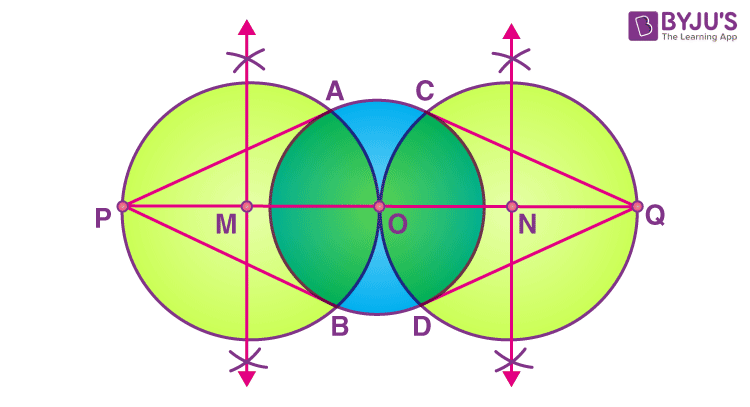Q. 4: Draw a circle with the help of a bangle. Take a point outside the circle. Construct the pair of tangents from this point to the circle.

Solution:

Steps of construction:

Step 1: Draw a circle with the help of a bangle.

Step 2: Draw two non-parallel chords such as AB and CD

Step 3: Draw the perpendicular bisector of AB and CD

Step 4: Take the centre as O where the perpendicular bisector intersects.

Step 5: To draw the tangents, take a point P outside the circle.

Step 6: Join the points O and P.

Step 7: Now draw the perpendicular bisector of the line PO and the midpoint is taken as M.

Step 8: Take M as centre and MO as radius, draw a circle.

Step 9: Let it intersect the circle at the points Q and R.

Step 10: Now join PQ and PR.

Therefore, PQ and PR are the required tangents.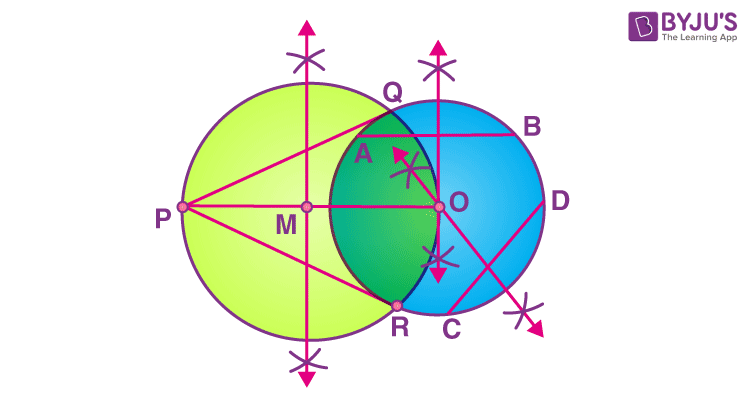Q. 5: Draw two concentric circles of radii 3 cm and 5 cm. Taking a point on the outer circle, construct the pair of tangents to the other. Measure the length of a tangent and verify it by actual calculation.

Solution:

Steps of construction:

Step 1: Draw a circle with centre O and radius 3 cm.

Step 2: Draw another circle with centre O and radius 5 cm.

Step 3: Take a point P on the circumference of a larger circle and join OP.

Step 4: Draw another circle such that it intersects the smallest circle at A and B.

Step 5: Join A to P and B to P.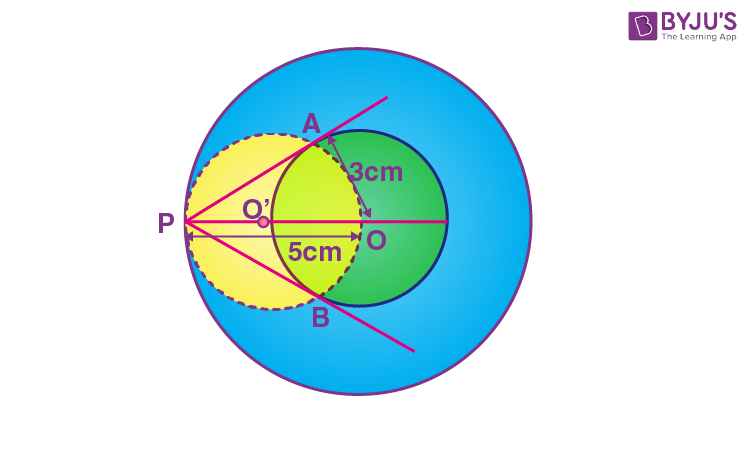Hence AP and BP are the required tangents.

Q.6: Draw a line segment AB of length 7 cm. Taking A as the centre, draw a circle of radius 3 cm and taking B as centre, draw another circle of radius 2 cm. Construct tangents to each circle from the centre of the other circle.

Solution:

Steps of Construction:

Step 1: Draw a line segment AB of 7 cm.

Step 2: Taking A and B as centres, draw two circles of 3 cm and 2 cm radius respectively.

Step 3: Bisect line AB. Let the midpoint of AB be C.

Step 4: Taking C as the centre, draw a circle of radius AC that intersects the two circles at points P, Q, R and S.

Step 5: Join BP, BQ, AS and AR.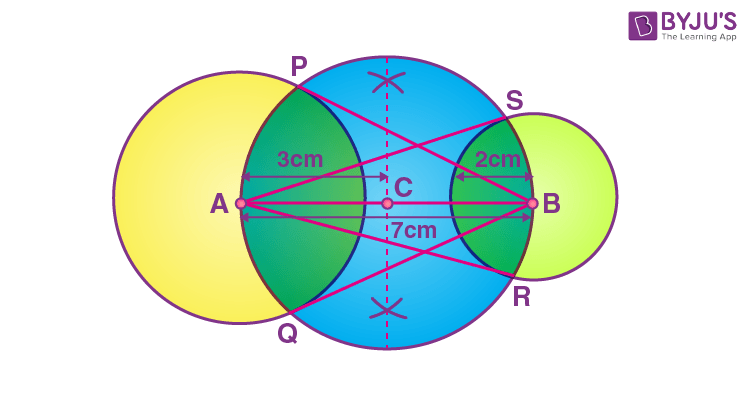PB, QB and RA and SA are the required tangents.

Q.7:  Construct an equilateral ΔABC with each side 5 cm. Then construct another triangle whose sides are 2/3 times the corresponding sides of ΔABC.

Solution:

Steps of construction:

Step 1: Draw a line segment BC = 5 cm.

Step 2: Taking B as centre and radius 5 cm, draw an arc.

Step 3: Now, taking C as centre and radius 5 cm, draw another arc meeting the previous arc at point A.

Step 4: Now join point AC and BC. Thus ΔABC is the required triangle.

Step 5: Draw a line BX such that ∠CBX is an acute angle and is opposite of vertex A.

Step 6: Along BX, mark 3 points B1, B2, B3 such that BB1 = B1B2 = B2B3.

Step 7: Now join B3 to C.

Step 8: Draw a line B2C’ || B3C

Step 9: Draw a line A’C’ parallel to AC.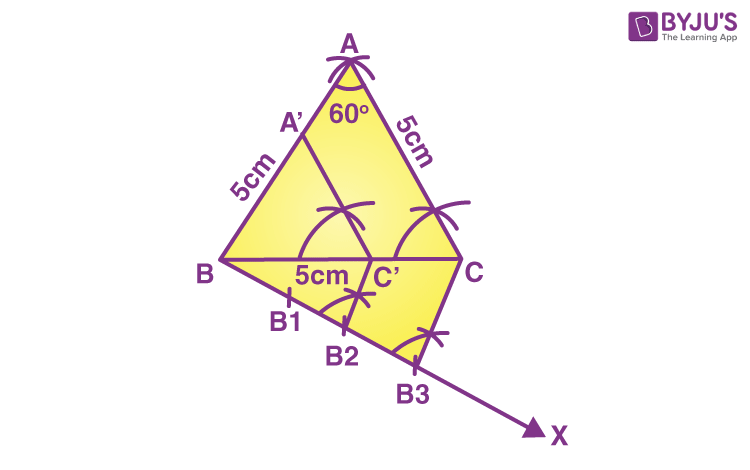Hence ΔBA’C’ is the required triangle.

Q.8: Construct a triangle ABC with side BC = 7 cm, ∠B = 45°, ∠A = 105°. Then construct another triangle whose sides are 3/4 times the corresponding sides of the ΔABC.

Solution:

In triangle ABC,

∠A + ∠B + ∠C = 180°

105° + 45° + ∠C = 180°

∠C = 180° – 150° = 30°

Steps of construction:

Step 1: Draw a line segment BC = 7 cm.

Step 2: At B, construct a right angle and bisect it such that ∠B = 45°.

Step 3: Construct an angle 30 degrees at C such that this line intersects the previous angle at A. Thus ΔABC is the required triangle.

Step 4: Draw a line BX such that ∠CBX is an acute angle and is opposite of vertex A.

Step 5: Along BX, mark 4 points B1, B2, B3, B4 such that BB1 = B1B2 = B2B3 = B3B4

Step 6: Now join B3 to C.

Step 7: Draw a line through B4 which is parallel to B3C such that it intersects the extended BC at C’.

Step 8: Draw a line A’C’ parallel to AC such that it meets the extended AB at A’.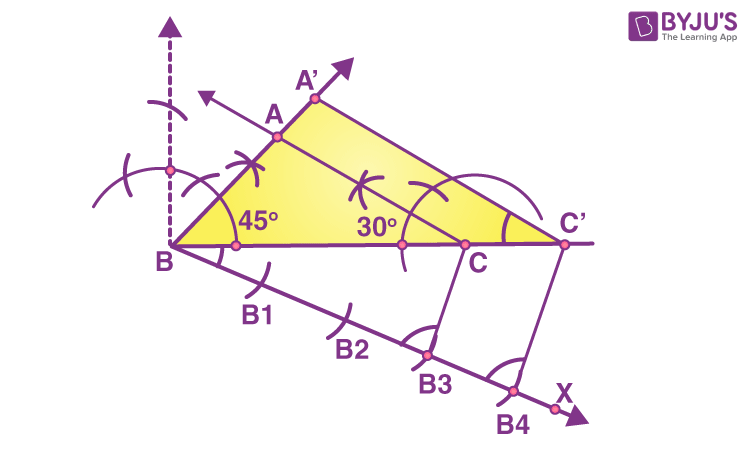Hence ΔBA’C’ is the required triangle similar to triangle ABC.

Q.9: Construct a ΔABC in which AB = 6 cm, ∠A = 30° and ∠B = 60°. Construct another ΔAB’C’ similar to ΔABC with base AB’ = 8 cm.

Solution:

Given, AB = 6 cm and AB’ = 8 cm

Scale factor = AB’/AB = 8/6 = 4/3

Steps of construction:

Step 1: Draw a line segment AB = 6 cm.

Step 2: At A and B, construct angles 30° and 60° respectively and let these lines intersect each other at C. Thus ΔABC is the required triangle.

Step 3: Draw a line AX such that ∠BAX is an acute angle and is opposite of vertex C.

Step 4: Along AX, mark 4 points A1, A2, A3, A4 such that AA1 = A1A2 = A2A3 = A3A4

Step 5: Now join A3B.

Step 6: Draw a line through A4 which is parallel to A3B such that it intersects the extended AB at B’.

Step 7: Draw a line B’C’ parallel to BC such that it meets the extended AC at C’.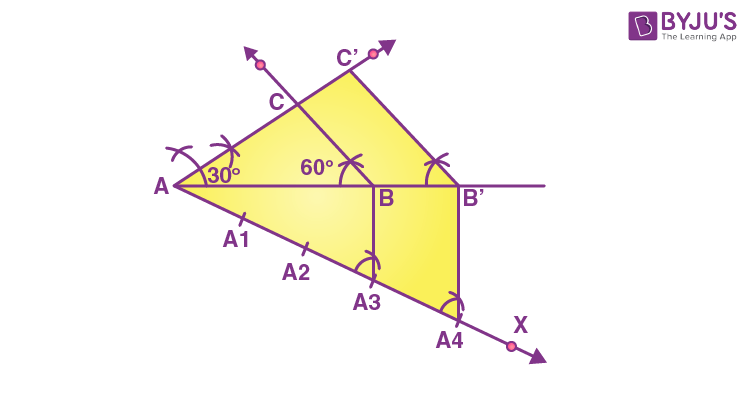Hence ΔBA’C’ is the required triangle similar to triangle ABC.

Q.10: Draw a circle of radius 4 cm. Draw two tangents to the circle inclined at an angle of 60° to each other.

Solution:

Given,

Angle between two tangents = 60

Angle at the centre = 2 × 60 = 120

Steps of construction:

Step 1: Draw a circle with a radius of 4 cm.

Step 2: Draw two radii OA and OB such that the angle between these radii is 120 degrees.

Step 3: Draw PA perpendicular to OP and PB perpendicular to OB (since the tangent is perpendicular to the tangent at the point of contact).

Step 4: PA and PB are the required tangents inclined to each other at an angle of 60°.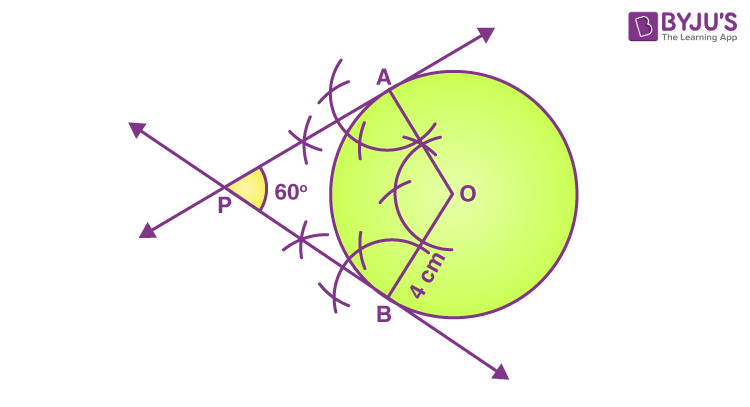### Practice Questions For Class 10 Maths Chapter 11 Constructions

1. Draw an isosceles triangle ABC in which AB = AC = 6 cm and BC = 5 cm. Construct a triangle PQR similar to ∆ ABC in which PQ = 8 cm. Also, justify the construction.
2. Let ABC be a right triangle in which AB = 6 cm, BC = 8 cm and ∠ B = 90°. BD is perpendicular to B on AC. The circle through B, C, D is drawn. Construct the tangents from A to this circle.
3. Draw a circle of radius 6 cm. From a point 10 cm away from its centre, construct the pair of tangents to the circle and measure their lengths.
4. Draw a triangle ABC with side BC = 6 cm, AB = 5 cm and ∠ABC = 60°. Then construct a triangle whose sides are 3/4 of the corresponding sides of the triangle ABC.
5. Draw a triangle PQR with side QR = 7 cm, angle Q = 45 degrees and Angle A = 105 degrees. After that, Construct a triangle whose sides are 3/4 of the corresponding sides of triangle ABC.
6. Use a bangle to draw a circle and take a point outside the circle. Now construct a pair of tangents from A to this circle.
7. Draw a line segment of length 7.6 cm and divide it in the ratio 5:8. Measure the two parts.
8. Draw a pair of tangents to a circle of radius 5 cm which is inclined to each other at an angle of 60 degrees.
9. Draw a triangle with sides 5 cm, 6 cm and 7 cm. Draw another triangle whose sides are 4/5 of the corresponding sides of the first triangle.
10. Construct a ΔABC in which AB = 6 cm, ∠A = 30° and ∠B = 60°. Construct another ΔAB’C’ similar to ΔABC with base AB’ = 8 cm.